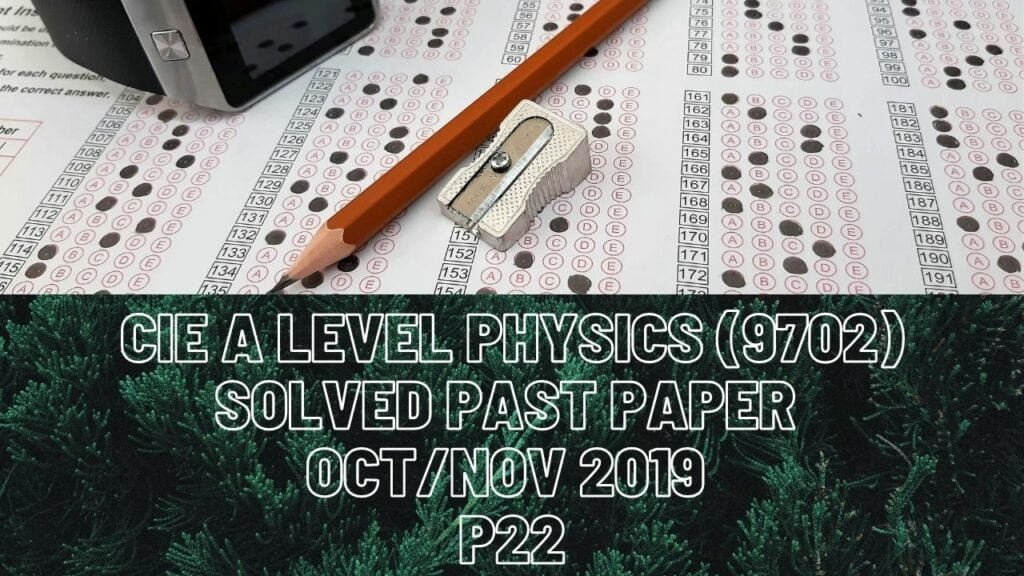A Level Physics### CIE A Level Physics Solved Past Paper Oct/Nov 2019 P22

2 biii) For the previous part of Q, the ball’s bottom had reached the beam of light and time interval for the ball to completely break the the beam of light would be when ball’s top also crosses that beam of light. The ball completely crossing light beam means it travels length of its diameter (0.080m), acceleration is due to free fall which is 9.81m/s^2 and the speed with which its bottom starts to cross the light beam is 2*9.81*0.280=5.5m/s. So now you have its initial velocity with which it enters, acceleration and the total distance it must cover before it completely crosses light beam.

c) When air resistance starts to act, resultant force on the ball decreases. When that decreases, acceleration also decreases and as a result time interval to cross light beam increases as they are inversely proportional.

3 biii) For a perfectly elastic collision, its momentum as well as kinetic energy is conserved.

cii) Block Y was initially at rest so, before head-on collision, it had the momentum of zero magnitude thus a horizontal line on the x-axis until t=20ms. In any case, whether elastic or inelastic collision, momentum should be conserved: In Fig.3.3, it can be seen that momentum of block X decreases after the collision so that of block Y should increase and thus will have a positive gradient. Force on block X is equal to force on block Y but in the opposite direction, so both should have equal gradients as (change in momentum/time taken) is resultant force and the time interval for the change in momentum should also be the same for both blocks. Since momentum change of block X covered 4 large boxes on the graph, momentum of block Y should also cover only 4 large boxes and should end a box sooner because it started from the x-axis.

4 bii) About point C, there are 3 forces acting on the left of the pivot out of which 2 cause anticlockwise moment and force at point B causes clockwise moment.

5 cii) Intensity maxima form only when incoming waves interfere constructively, there is no phase change between the two waves and path difference may be in whole numbers like 1?, 2?, 3?…

iv) Next intensity maximum forms when path difference is again in whole numbers like ?, 2? or 3?. Here wavelength has been increased which means at next maximum point, path difference would be of 3? since the last time it was 4?. Path difference could decrease from 4? to 7/2? but that path difference won’t form maximum point.

6 ci) Emf of battery is equal to voltage across either diode and resistor X or across resistor Y.

ii) Current divides in parallel combination so current that enters second loop is (9.3-7.5=1.8mA) and voltage across resistor Y is equal to emf of the battery.

iv) If Emf of the battery is set as 0.5V that means current of x should flow through components X and diode so that voltage in the first loop is 0.5V. Through fig.6.1, we can find out that at which value of current do we get a sum of 0.5V of p.d across both components X and diode. At I=2.5mA, voltage across X is 0.1V and that across diode is 0.4V that makes up a total p.d of 0.5V.